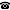# 2011 seminar talk: Weak Reflection Principle, Saturation of the ideal NS and Diamonds in two cardinal version

Talk held by Victor Torres Perez (KGRC) at the KGRC seminar on 2011-11-17.

### Abstract

We prove that the Weak Reflection Principle and the saturation of the ideal NS together imply $$\Diamond_{[\lambda]^{\omega_1}}$$, for all regular $$\lambda\geq\aleph_2$$. Even more, we get $$\Diamond_{[\lambda]^{\omega_1}}$$ concentrated on $$x\in[\lambda]^{\omega_1}$$ such that $$\sup(x)$$ has uncountable cofinality.

Kurt Gödel Research Center for Mathematical Logic. Währinger Straße 25, 1090 Wien, Austria.+43-1-4277-50501. Last updated: 2010-12-16, 04:37.